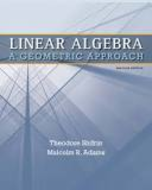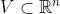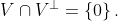Linear Algebra: A Geometric Approach - 2 Edition - Chapter 3.1 - Problem 10
Register Now

Join StudySoup

Get Full Access to Linear Algebra: A Geometric Approach - 2 Edition - Chapter 3.1 - Problem 109781429215213

# Let V Rn be a subspace. Show that V V = {0}

Linear Algebra: A Geometric Approach | 2nd Edition

Problem 10

Let $$V \subset \mathbb{R}^n$$ be a subspace. Show that $$V \cap V^{\perp}=\{0\}$$.

Accepted Solution
Step-by-Step Solution:

Problem 10

Letbe a subspace. Show thatStep-by-step solution

Step 1 of 2

Given thatis a subspace of###### Chapter 3.1, Problem 10 is Solved

Step 2 of 2

Unlock Textbook Solution